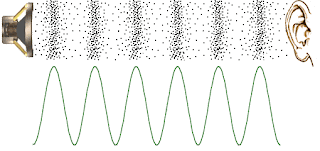# bjorg

## Sunday, July 21, 2013

### Peak Meters, dBFS and HeadroomThe level meter from audiofile engineering's spectre program accurately shows peak values in dBFS
Level meters are one of the most basic features of digital audio software. In software, they are very often implemented as peak meters, which are designed to track the maximum amplitude of the signal. Other kinds of meters, such as VU meters, are often simulations of analog meters. Loudness meters, which attempt to estimate our perception of volume rather than volume itself, are also becoming increasingly common. You may also come across RMS and average meters. In this post, I'm only going to talk about peak meters.

### Peak Meters

Peak meters are useful in digital audio because they show the user information that is closely associated with the limits of the medium and because they are efficient and easy to implement. Under normal circumstances, we can expect peak meters to correspond pretty well with our perception of volume, but not perfectly. The general expectation users have when looking at peak meters is that if a signal goes above a certain level at some point, that level should be indicated on the meters. In other words, if the signal goes as high as, say -2 dBFS, over some time period, then someone watching the peak meter during that time will see the meter hit the -2 dBFS mark (see below for more on dBFS). Many peak meters have features such as "peak hold" specifically designed so that the user does not need to stare at the meter.

Beyond that, there are rarely any specifics. Some peak meters show their output linearly, some show their output in dB. Some use virtual LEDs, some a bar graph. In general, if there is a numeric readout or units associated with the meter, the unit should be dBFS.

Now that we know the basics of peak meters, let's figure out how to implement them.

### Update Time

Peak meters should feel fast and responsive. However, they don't update instantly. In software, it is not uncommon to have audio samples run at 44100 samples per second while the display refreshes at only 75 times per second, so there is absolutely no point in showing the value of each sample (not to mention the fact that our eyes couldn't keep up). Clearly we need to figure out how to represent a large number of samples with only one value. For peak meters, we do this as follows:

1. Figure out how often we want to update. For example, every 100 ms (.1s) is a good starting point, and will work well most of the time.
2. Figure out how many samples we need to aggregate for each update. If we are sampling at 44100 Hz, a common rate, and want to update every .1s, we need N = 44100 * .1 = 4410 samples per update.
3. Loop on blocks of size N. Find the peak in each block and display that peak. If the graphics system does not allow us to display a given peak, the next iteration should display the max of any undisplayed peaks.

### Finding the PeakSound is created by air pressure swing both above below the mean pressure.
Finding the peak of each block of N samples is the core of peak metering. To do so, we can't simply find the maximum value of all samples because sound waves contain not just peaks, but also troughs. If those troughs go further from the mean than the peaks, we will underestimate the peak.

The solution to this problem is simply to take the absolute value of each sample, and then find the max of those absolute values. In code, it would look something like this:

float max = 0;
for( int i=0; i<buf.size(); ++i ) {
const float v = abs( buf[i] )
if( v > max )
max = v;
}

At the end of this loop, max is your peak value for that block, and you can display it on the meter, or, optionally, calculate its value in dBFS first.

(For a more complete and less "arm wavy" intro to decibels, try here or here.) The standard unit for measuring audio levels is the decibel or dB. But the dB by itself is something of an incomplete unit, because, loosely speaking, instead of telling you the amplitude of something, dB tells you the amplitude of something relative to something else. Therefore, to say something has an amplitude of 3dB is meaningless. Even saying it has an amplitude of 0dB is meaningless. You always need some point of reference. In digital audio, the standard point of reference is "Full Scale", ie, the maximum value that digital audio can take on without clipping. If you are representing your audio as a float, 0 dB is nominally calibrated to +/- 1.0. We call this scale dBFS. To convert the above max value (which is always positive because it comes from an absolute value) to dBFS use this formula:

dBFS = 20 * log10(max);

You may find it odd that the loudest a signal can normally be is 0 dBFS, but this is how it is. You may find it useful to think of dBFS as "headroom", ie, answering the question "how many dB can I add to the signal before it reaches the maximum?" (Headroom is actually equal to -dBFS, but I've often seen headroom labeled as dBFS when the context makes it clear.)

#### 1 comment:

1.May 2018 and the link doesn't work. the program is nowhere to be found.